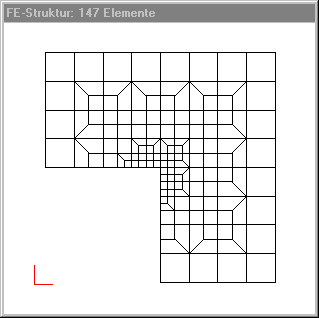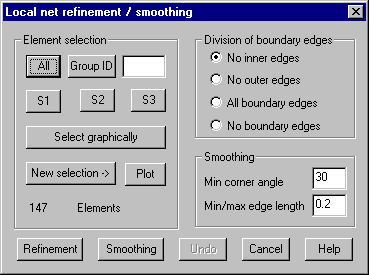Net refinement: Local net refinement by dividing individual elements, respectively smoothing of a plane FE net.

This command is only applicable on plane FE nets consisting of only triangular and quadrilateral elements.

Refinement: Pressing this button, triangular elements are subdivided into 3 and quadrilateral elements are subdivided into 4 elements. One additional node is created in the inner of the elements and on the element edges of selected elements. In reference to the element edges that belong to the border of the selected area, it is distinguished between edges that belong to the border of the entire structure and element edges that do not belong to this border. It has to be specified, which of these two kinds of border edges should be subdivided.

Hint: The number of elements belonging to an element row next the inner border of the selected area should be even, so that only quadrilateral elements can be generated (see following figure).Smoothing: Clicking this button, the selected area of the net is smoothed by using following algorithm: Successively, for each node in the inner of the selected area, the directly connected edges are determined and the coordinates of the node are corrected to the mean value of all nodes of these connected edges. The algorithm is used several times for each node. For smoothing a smallest corner angle and a ration for smallest to largest edge length of elements must be given. Correction of node coordinates is not done if the angle of element corners gets smaller than the given angle or greater than 180 -  given angle, or if edge length gets to small.

Following dialog shows the available options:Division of boundary edges

No inner edges: Element edges belonging to the border of the selected area but not to the border of the entire structure are not subdivided.

No outer edges: Element edges belonging to the border of the entire structure are not subdivided.

All boundary edges: All element edges belonging to the border of the selected area are subdivided.

No boundary edges: No element edges belonging to the border of the selected area are subdivided.

Smoothing

Min corner angle: An angle must be given in the input field, in case of smoothing the net, all angles in the corners of the elements are checked against this angle, and correction of the coordinates of some nodes is not done if an angle in the element corners gets less than the given value.

Min/max edge length: The ratio of smallest to largest edge length of elements must be given in the input field. In case of smoothing the net, all edge length of the elements are checked against this value, and correction of the coordinates of some nodes is not done if the ratio of edge length gets less than this value.

Element selection

Select the elements that belong to the area that is to be refined or that shall be smoothed.

Undo

Clicking this button will undo the refinement respectively smoothing